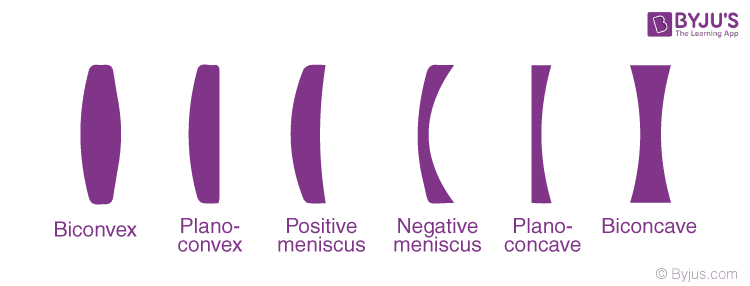Checkout JEE MAINS 2022 Question Paper Analysis : Checkout JEE MAINS 2022 Question Paper Analysis :

# Lenses In Optics

## What are Lenses?

A lens is a transmissive optical device that focuses or disperses light beams by means of refraction. A simple lens consists of a single piece of transparent material, while compound lenses consist of several simple lenses arranged along with a common axis. A lens can focus light to form an image, unlike a prism, which refracts light without focusing.

### Mirrors Vs Lens

The most apparent distinction between mirrors and lenses are: mirrors reflect light rays (light bounces back) while light rays are refracted (pass-through) through a lens. A mirror will have only one focal point, which is in front of the mirror. A lens has two focal points each on either side. In the table below, let us look at more differences between mirror and lens.

 Mirror Lens A mirror is a glass surface with a silvery backing, that produces an image through reflection. A lens is a transparent substance of glass or plastic, bound by two surfaces, whose at least one surface is curved. It can either be plane or curved It is usually curved The working principle of the mirror is the law of reflection The working principle of the mirror is the law of refraction

In the next section, let us discuss the different types of lenses.

## Types Of Lenses

The classification of a lens depends on how the light rays bend when they pass through the lens. The two main types of lenses are:

• Convex Lens (Converging)
• Concave Lens (Diverging)

Convex lenses are thick in the middle and thinner at the edges. A concave lens is flat in the middle and thicker at the edges. A convex lens is also known as the converging lens as the light rays bend inwards and converge at a point which is known as focal length. On the other hand, the concave lens is also known as a diverging lens because it bends the parallel light rays outward and diverges them at the focal point.

Simple lens and compound lens are the two classifications of lenses. Simple lenses are different from compound lenses based on their surface of curvature. Following are the different types of simple lenses.Compound lenses are those constructed out of a combination of different simple lenses. The lens types used to build a compound lens may have different refractive indices and other properties. The placement of lenses are such that they lie on a single axis, and properties like focal length are calculated again for the new compound lens.

Other Lens Types are:

• Cylindrical – curvature in one direction
• Fresnel – narrow ring-like surface
• Lenticular – a group of micro-lenses
• Gradient Index – flat surfaces but with varying refractive indices
• Axicon – conical surface

What if we need to find out the exact position of an image with respect to the lens’ position? Do we need to draw a diagram every time? This video defines the Lens Formula, Magnification and Power for lenses and how simple math can be used to make life easy. It also expands on concepts taught in previous classes and defines the power of a lens.### Applications Of Lens

What do magnifying lens, your eye and binoculars have in common? They all use convex lenses. And have any of you wondered why people look so weird when you see them through a peephole? That’s because the glass used there isn’t a normal one. It is a concave lens. Convex lenses are used to correct farsighted vision problems.

Similarly, concave lenses are used to correct nearsightedness. Compound microscopes and telescopes use both convex and concave lenses. Using combinations of convex lenses may create blurry images. A concave eyepiece is used to correct this problem. Even in cameras at times a combination of concave and convex lenses are used. The use of concave lenses is essential in lasers. The actual light ray is highly specific, which may damage equipment where it is used (like CDs and scanners). The diverging lens rectifies this issue. Concave lenses also find application in flashlights where the light beam diverges for broader area coverage.

Learn about lenses and their real-life applications with experienced tutors here at BYJU’S.

## Frequently Asked Questions – FAQs

### What is optics?

Optics is a discipline of physics that deals with the properties and behaviour of light, including its interplay with matter and the creation of devices that use it. In other words, optics explains the behaviour of infrared, visible and ultraviolet light.

### What is a lens?

A lense is a transmissive device which disperses or focuses light beams by means of refraction.

### What are the main two types of lenses?

Concave lenses and convex lenses are the main two types of lenses.

### What is the main difference between a lens and a mirror?

The main difference between a lens and a mirror is that light rays are refracted through a lens while mirrors reflect or bounce back light rays.

### What are the important uses of lenses?

Lenses are employed in different imaging tools like cameras, binoculars, and telescopes. They are also extensively used for correcting vision defects such as hypermetropia and myopia.

Test your knowledge on Lenses in optics

#### 1 Comment

1. D.Devi Harshitha

Nice